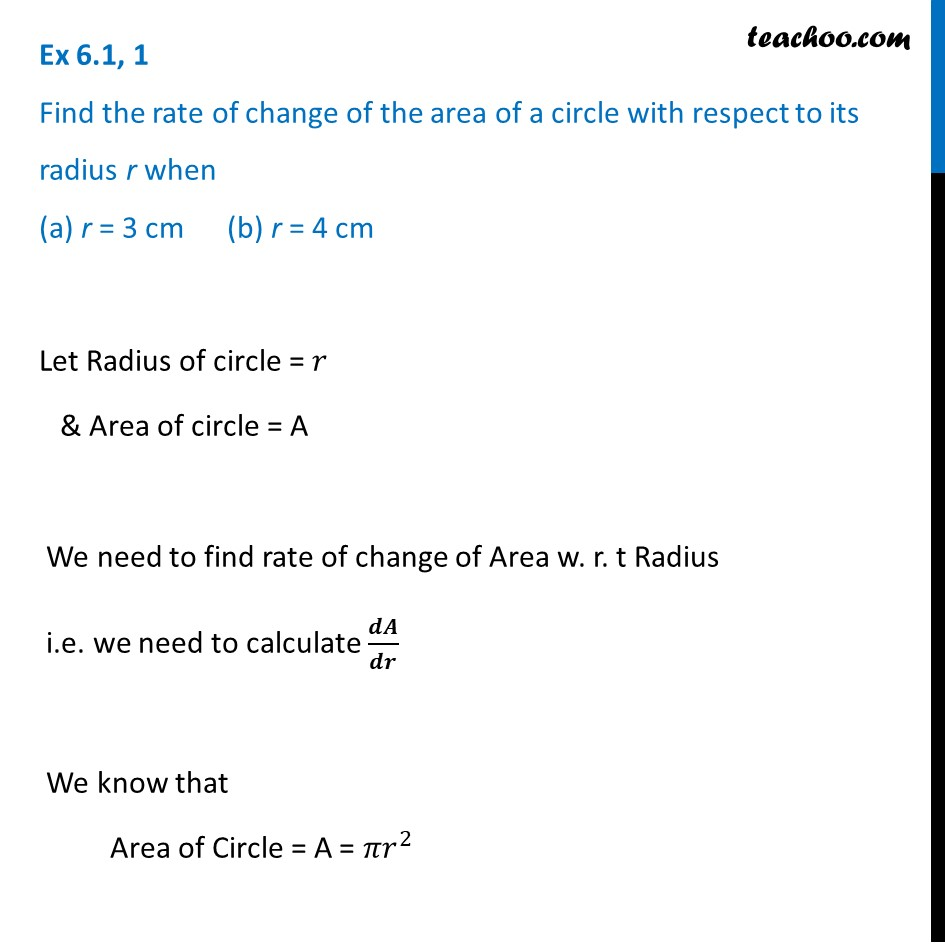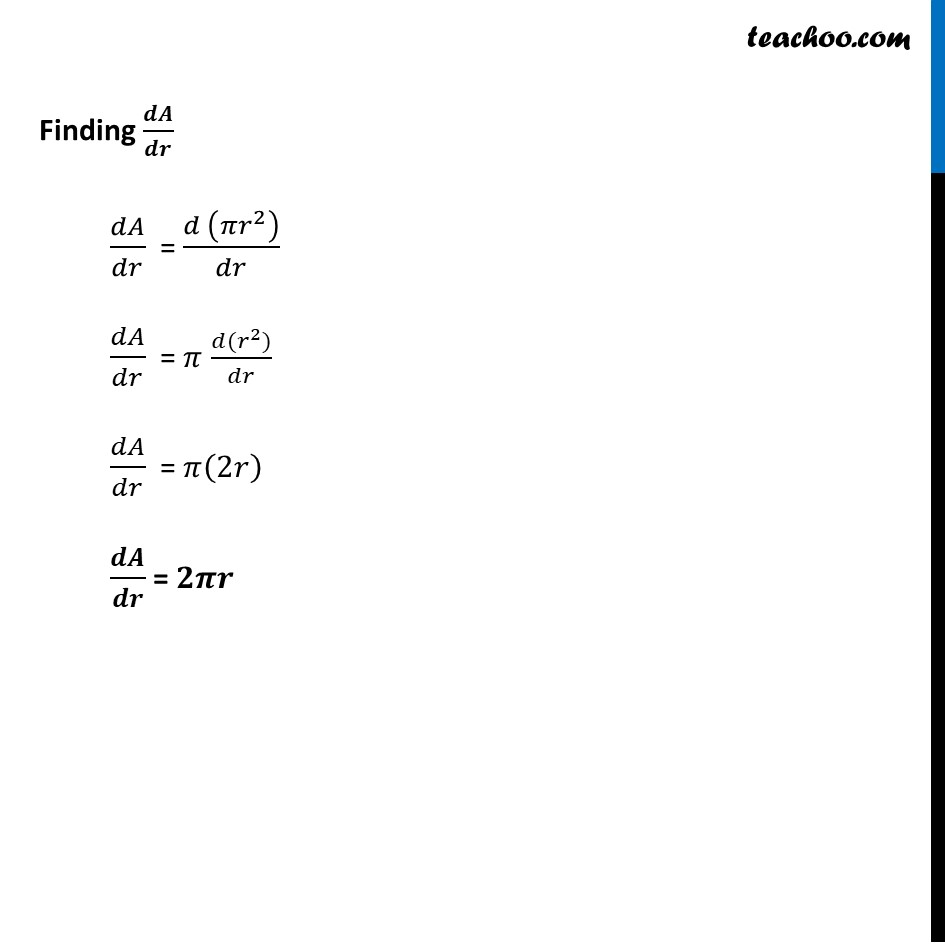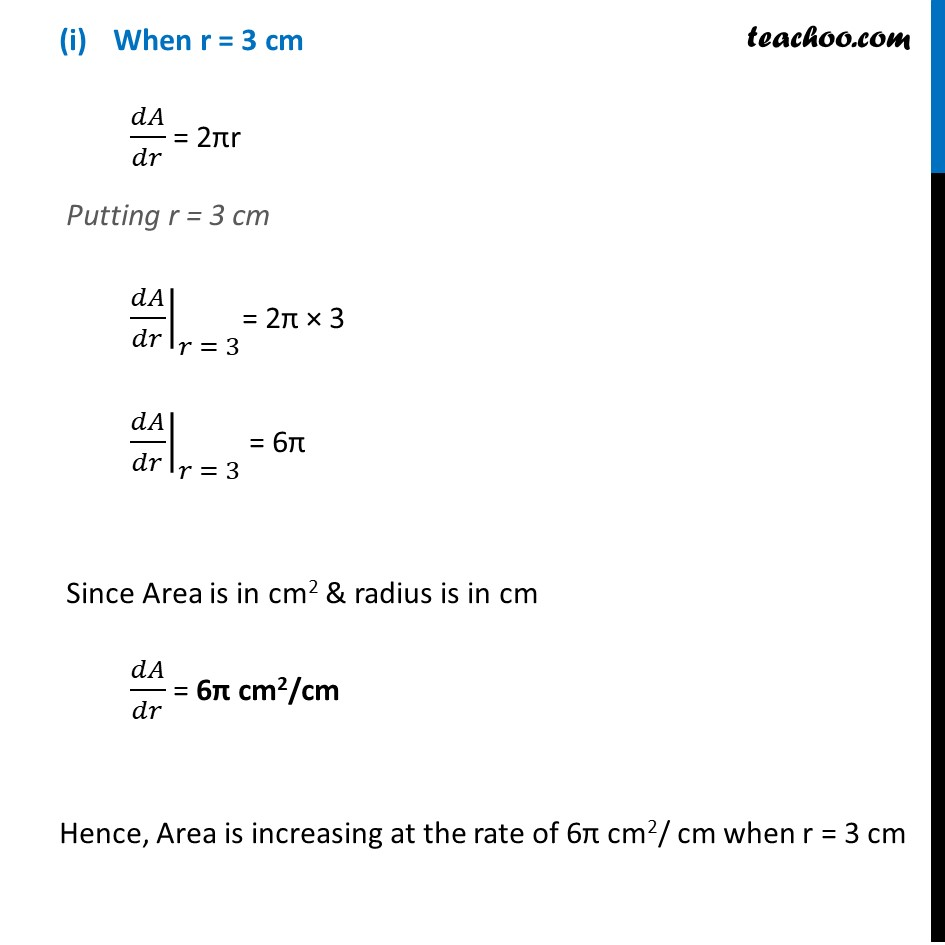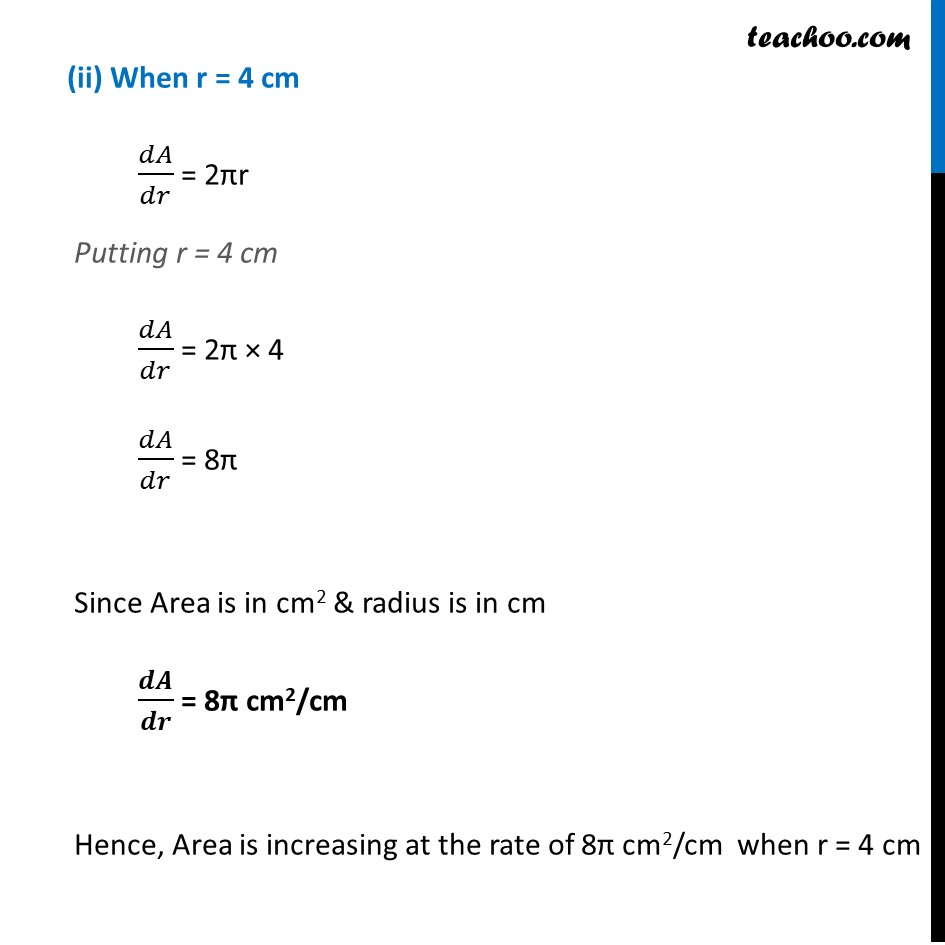1. Chapter 6 Class 12 Application of Derivatives (Term 1)
2. Serial order wise
3. Ex 6.1

Transcript

Ex 6.1, 1 Find the rate of change of the area of a circle with respect to its radius r when (a) r = 3 cm (b) r = 4 cmLet Radius of circle = 𝑟 & Area of circle = A We need to find rate of change of Area w. r. t Radius i.e. we need to calculate 𝒅𝑨/𝒅𝒓 We know that Area of Circle = A = 〖𝜋𝑟〗^2 Finding 𝒅𝑨/𝒅𝒓 𝑑𝐴/𝑑𝑟 = (𝑑 (〖𝜋𝑟〗^2 ))/𝑑𝑟 𝑑𝐴/𝑑𝑟 = 𝜋 (𝑑〖(𝑟〗^2))/𝑑𝑟 𝑑𝐴/𝑑𝑟 = 𝜋(2𝑟) 𝒅𝑨/𝒅𝒓 = 𝟐𝝅𝒓 When r = 3 cm 𝑑𝐴/𝑑𝑟 = 2πr Putting r = 3 cm ├ 𝑑𝐴/𝑑𝑟┤|_(𝑟 = 3)= 2π × 3 ├ 𝑑𝐴/𝑑𝑟┤|_(𝑟 = 3) = 6π Since Area is in cm2 & radius is in cm 𝑑𝐴/𝑑𝑟 = 6π cm2/cm Hence, Area is increasing at the rate of 6π cm2/ cm when r = 3 cm (ii) When r = 4 cm 𝑑𝐴/𝑑𝑟 = 2πr Putting r = 4 cm 𝑑𝐴/𝑑𝑟 = 2π × 4 𝑑𝐴/𝑑𝑟 = 8π Since Area is in cm2 & radius is in cm 𝒅𝑨/𝒅𝒓 = 8π cm2/cm Hence, Area is increasing at the rate of 8π cm2/cm when r = 4 cm

Ex 6.1

Chapter 6 Class 12 Application of Derivatives (Term 1)
Serial order wise